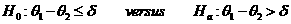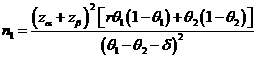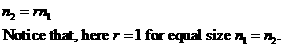# Sample Size Calculator: Two Parallel-Sample Proportions

Hypothesis: One-Sided Non-Inferiority / SuperiorityData Input: (Help) (Example)

 Input Results α β n1 θ1 n2 θ2 N δ r

Note:

 Variables Descriptions α One-sided significance level 1-β Power of the test θ1 Expected success proportions of sample one θ2 Expected success proportions of sample two δ δ>0 for the superiority margin or δ<0 for the non-inferiority margin r Ratio of sample size of group two to group one n1 Sample size of each sample one n2 Sample size of each sample two N Total Sample size

Help Aids Top

Application: This procedure is used to test non-inferiority and superiority that can be unified by the following hypothesis:Where δ is the superiority (δ > 0) or non-inferiority margin (δ < 0).

Procedure:

1. Enter

a)      value of α, the probability of type I error

b)      value of β, the probability of type II error

c)      value of θ1, a true mean response rate of a test drug

d)     value of θ2, the true mean response rates of a control drug

e)      value of δ, the superiority for δ>0 or non-inferiority for δ<0

f)       value of r, allocation ratio, n2/n1.

1. Click the button “Calculate” to obtain result sample size of each group n.

Formula:(*)Notations:

α:               The probability of type I error (significance level) is the probability of rejecting the true null hypothesis.

β:               The probability of type II error (1 – power of the test) is the probability of not rejecting the false null hypothesis.

θ12:         The difference between the true mean response rates of a group1 (i.e., a test drug (θ1) and group2 (i.e., a control (θ2))).

r:                The allocation ratio n2/n1. i.e., r=1 for equal allocation.

δ:               The superiority for δ>0 or non-inferiority for δ<0. When δ>0, the rejection of the null hypothesis indicates the superiority of the test drug over the control. When δ<0, the rejection of the null hypothesis indicates the non-inferiority of the test drug against the control.

Examples

Example 1: Suppose it is of interest to establish non-inferiority of the test drug as compared to the active control agent. Considering of the difference less than 10% is of no clinical importance. Thus, the non-inferiority margin is selected to be δ=-0.1. Now given that the true mean cure rates of the treatment agents and the active control are θ1=85% and θ2=65%, respectively. Then, by (*), the required sample size with equal allocation (r=1) to achieve an 80% power (β=0.2) at α=0.05 can be determined by n1 = n2 = 25.

Similarly, for a pharmaceutical company may want to show superiority of the test drug over the active control agent. Assume the superiority margin is δ=0.05. According to (*), we have the sample sizes with equal allocation are n1 = n2 = 98. Notice that θ1=0.85 and θ2=0.65 here.

Reference:

1. Casagrande, Pike and Smith (1978), Biometrics 34: 483-486.
2. Chow, Shao and Wang, Sample Size Calculations In Clinical Research, Taylor & Francis, NY. (2003) Page 88.
3. Flesis J.L., Statistical Methods for Rates and Proportions (2nd edition). Wiley: New York, 1981.

Top